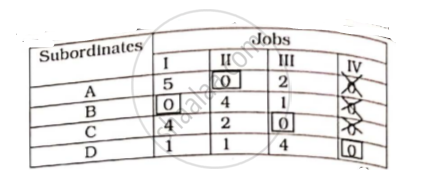# Solve the Following Assignment Problem to Minimize the Cost: - Mathematics and Statistics

Sum

Solve the following assignment problem to minimize the cost:

 Persons Jobs I II III A 7 3 5 B 2 7 4 C 6 5 3 D 3 4 7

#### Solution

Number of columns ≠  Number of rows

∴  Given problem is unbalanced

Step 1:  For making it balanced, we add dummy job(iv) with cost zero

Step 2: Minimum elements of each row is subtracted from every element of that row. Resultant matrix is same.

Step 3: Minimum element in each column is subtracted from every element in that column.

 Subordinates Jobs I II III IV A 5 0 2 0 B 0 4 1 0 C 4 2 0 0 D 0 1 4 0

Zero element are covered with minimum number of straight lines:

 Subordinates Jobs I II III IV A 5 0 2 0 B 0 4 1 0 C 4 2 0 0 D 0 1 4 0

Number of lines covering all zero is equal to number of rows/columns. The optimal solution has been reached. · Optimal assignment can be made as follows:

 Subordinates Jobs I II III IV A 5 0 2 0 B 0 4 1 0 C 4 2 0 0 D 1 1 4 0∴ Optimal assignment is obtained.

 Subordinates Jobs Effectiveness A II 3 B I 2 C III 3 D IV 0

The total (minimum) effectlveness = 3 + 2 + 3 + 0 = 8.

Concept: Maxima and Minima
Is there an error in this question or solution?